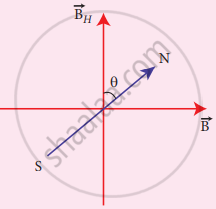Tamil Nadu Board of Secondary EducationHSC Science Class 12

# What is tangent law? Discuss in detail. - Physics

What is tangent law? Discuss in detail.

#### Solution

Tangent law:

When a magnetic needle or magnet is freely suspended in two mutually perpendicular uniform magnetic fields, it will come to rest in the direction of the resultant of the two fields.

Explanation:

Let B be the magnetic field produced by passing a current through the coil of the tangent Galvanometer and BH be the horizontal component of the earth’s magnetic field. Under the action of two magnetic fields, the needle comes to rest making angle θ with BH, such thatResultant position of pivoted needle

tan θ = "B"/"B"_"H"

B = BH tan θ ……. (1)

When no current is passed through the coil, the small magnetic needle lies along the horizontal component of Earth’s magnetic field. When the circuit is switched ON, the electric current will pass through the circular coil and produce a magnetic field. Now there are two fields that are acting mutually perpendicular to each other. They are:

1. the magnetic field (B) due to the electric current in the coil acting normal to the plane of the coil.
2. the horizontal component of Earth’s magnetic field (BH)
Because of these crossed fields, the pivoted magnetic needle deflects through an angle θ. From tangent law, B = BH tan θ When an electric current is passed through a circular coil of radius R having N turns, the magnitude of the magnetic field at the center is
B = mu_0 "NI"/"2R"    ....(2)
From equation (1) and equation (2), we get
mu_0 "NI"/"2R"= BH tan θ
The horizontal component of Earth’s magnetic field can be determined as
B = mu_0 "NI"/(2 "R" tan theta) in tesla ……. (3)
Concept: Biot - Savart Law
Is there an error in this question or solution?
Chapter 3: Magnetism and magnetic effects of electric current - Evaluation [Page 192]

#### APPEARS IN

Tamil Nadu Board Samacheer Kalvi Class 12th Physics Volume 1 and 2 Answers Guide
Chapter 3 Magnetism and magnetic effects of electric current
Evaluation | Q III. 9. | Page 192
Share